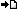RegisterMember LoginForgot Password ?? PHP , ASP , ASP.NET, VB.NET, C#, Java , jQuery , Android , iOS , Windows Phone

## Registered : 109,019

 HOME > PHP Manual > usort - Sort an array by values using a user-defined comparison function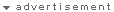## ลองใช้ค้นหาข้อมูล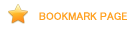# usort

(PHP 4, PHP 5)

usortSort an array by values using a user-defined comparison function

### Description

bool usort ( array &\$array , callback \$cmp_function )

This function will sort an array by its values using a user-supplied comparison function. If the array you wish to sort needs to be sorted by some non-trivial criteria, you should use this function.

Note: If two members compare as equal, their order in the sorted array is undefined.

Note: This function assigns new keys to the elements in array . It will remove any existing keys that may have been assigned, rather than just reordering the keys.

### Parameters

array

The input array.

cmp_function

The comparison function must return an integer less than, equal to, or greater than zero if the first argument is considered to be respectively less than, equal to, or greater than the second.

### Return Values

Returns TRUE on success or FALSE on failure.

### ChangeLog

Version Description
4.1.0 A new sort algorithm was introduced. The cmp_function doesn't keep the original order for elements comparing as equal.

### Examples

Example #1 usort() example

``` <?phpfunction cmp(\$a, \$b){    if (\$a == \$b) {        return 0;    }    return (\$a < \$b) ? -1 : 1;}\$a = array(3, 2, 5, 6, 1);usort(\$a, "cmp");foreach (\$a as \$key => \$value) {    echo "\$key: \$value\n";}?> ```

The above example will output:

```0: 1
1: 2
2: 3
3: 5
4: 6

```

Note: Obviously in this trivial case the sort() function would be more appropriate.

Example #2 usort() example using multi-dimensional array

``` <?phpfunction cmp(\$a, \$b){    return strcmp(\$a["fruit"], \$b["fruit"]);}\$fruits["fruit"] = "lemons";\$fruits["fruit"] = "apples";\$fruits["fruit"] = "grapes";usort(\$fruits, "cmp");while (list(\$key, \$value) = each(\$fruits)) {    echo "\\$fruits[\$key]: " . \$value["fruit"] . "\n";}?> ```

When sorting a multi-dimensional array, \$a and \$b contain references to the first index of the array.

The above example will output:

```\$fruits: apples
\$fruits: grapes
\$fruits: lemons

```

Example #3 usort() example using a member function of an object

``` <?phpclass TestObj {    var \$name;    function TestObj(\$name)    {        \$this->name = \$name;    }    /* This is the static comparing function: */    static function cmp_obj(\$a, \$b)    {        \$al = strtolower(\$a->name);        \$bl = strtolower(\$b->name);        if (\$al == \$bl) {            return 0;        }        return (\$al > \$bl) ? +1 : -1;    }}\$a[] = new TestObj("c");\$a[] = new TestObj("b");\$a[] = new TestObj("d");usort(\$a, array("TestObj", "cmp_obj"));foreach (\$a as \$item) {    echo \$item->name . "\n";}?> ```

The above example will output:

```b
c
d

```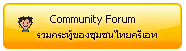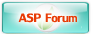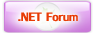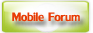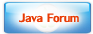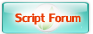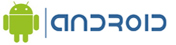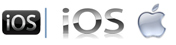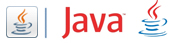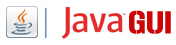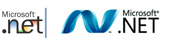Free Tutorial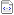สอน Google Maps Apiสอน Windows Serviceสอน Entity Frameworkสอน Androidสอน Java เขียน JavaJava GUI Swingสอน JSP (Web App)iOS (iPhone,iPad)Windows PhoneWindows AzureWindows StoreLaravel FrameworkYii PHP Frameworkสอน jQueryสอน jQuery กับ Ajaxสอน PHP OOP (Vdo)Ajax Tutorials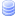SQL Tutorialsสอน SQL (Part 2)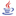JavaScript TutorialJavascript TipsVBScript TutorialVBScript ValidationMicrosoft Access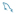MySQL Tutorials-- Stored ProcedureMariaDB Database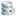SQL Server TutorialSQL Server 2005SQL Server 2008SQL Server 2012-- Stored ProcedureOracle Database-- Stored ProcedureSVN (Subversion)แนวทางการทำ SEOปรับแต่งเว็บให้โหลดเร็วลองค้นหาสิ่งที่ต้องการLoad balance : Server 04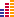© www.ThaiCreate.Com. 2003-2023 All Rights Reserved. ไทยครีเอทบริการ จัดทำดูแลแก้ไข Web Application ทุกรูปแบบ (PHP, .Net Application, VB.Net, C#) [Conditions Privacy Statement] ติดต่อโฆษณา 081-987-6107 อัตราราคา คลิกที่นี่